unique random number between 1,10 in single array formula

The Randbetween function may generate duplicate value. if we used the formula

=Randbetween(1,10) it will generate random numbers between 1 and 10, if we applied the formula in ten cells there is a possibility the formula may generate duplicate value in the ten cells. To avoid this you can use the following formula

=ARRAYFORMULA(LARGE(ROW(\$1:\$10)*NOT(COUNTIF(\$A\$1:A1, ROW(\$1:\$10))), RANDBETWEEN(1,11-ROW(A1))))

Copy cell A2 and paste down as far as needed.

Explaining the alternative array formula in cell A2

Step 1 – Create an array
ROW(\$1:\$10) creates this array {1, 2, 3, 4, 5, 6, 7, 8, 9, 10}

Step 2 – Create a criterion to avoid duplicate numbers
COUNTIF(\$A\$1:A1, ROW(\$1:\$10)) makes sure no duplicate numbers are created. The formula has both absolute and relative cell references (\$A\$1:A1). When the formula are copied down to cell A3 the cell reference changes to \$A\$1:A2. The value in cell A2 can´t be randomly selected again.
In cell A2, COUNTIF(\$A\$1:A1, ROW(\$1:\$10)) creates this array: {0, 0, 0, 0, 0, 0, 0, 0, 0, 0}

Step 3 – Create a new dynamic array
ROW(\$1:\$10)*NOT(COUNTIF(\$A\$1:A1, ROW(\$1:\$10))) creates this array in cell A2: {1, 2, 3, 4, 5, 6, 7, 8, 9, 10}
If the array formula  randomly selects the number 2 in cell A2, the formula in cell A3 creates this array: {1, 0, 3, 4, 5, 6, 7, 8, 9, 10}
Number 2 can´t be selected anymore.

Step 4 – Calculate the number range in Randbetween(bottom, top)
The bottom value is always 1. The top value changes depending on current cell.
In cell A2 the top value is 10.
In cell A3 the top value is 9
and so on..
Formula in cell A2: 11-ROW(A1) equals 10. (11-1=10)
Formula in cell A3: 11-ROW(A2) equals 9. (11-2=9)
and so on..

Step 5 – Create a random number
=LARGE(ROW(\$1:\$10)*NOT(COUNTIF(\$A\$1:A1, ROW(\$1:\$10))), RANDBETWEEN(1,11-ROW(A1)))
RANDBETWEEN(1,11-ROW(A1))
becomes
RANDBETWEEN(1,11-1)
becomes
RANDBETWEEN(1,10)
and returns a random number between 1 and 10.

Step 6 – Select a random number in array
=LARGE(ROW(\$1:\$10)*NOT(COUNTIF(\$A\$1:A1, ROW(\$1:\$10))), RANDBETWEEN(1,11-ROW(A1)))
becomes
=LARGE({1, 2, 3, 4, 5, 6, 7, 8, 9, 10}, RANDBETWEEN(1,10))
becomes
=LARGE({1, 2, 3, 4, 5, 6, 7, 8, 9, 10}, random_number) and returns a random number between 1 and 10.

SUMPRODUCT

The SUMPRODUCT function returns the sum of the products of corresponding numbers in one or more arrays.

SUMPRODUCT (array 1, array 2, …array 30)

array1: A array  of cells. array 1  is a reference to a single range of cells containing values of any type. If string or Boolean values
array 2,……. array 30: Optionally include one or more additional array ( ranges) of cells. The ranges must all have the same dimensions.

Usage Notes
The SUMPRODUCT function multiplies the corresponding numbers in each range and then sums each of the products. If only one range is specified, SUMPRODUCT returns the sum of the range.

Traditional Use of SUMPRODUCT

In it’s traditional form or classic form, SUMPRODUCT multiplies each value in one array by the corresponding value in another array, and returns the summed result. As an example, if cells A1:A3 contain the values 1,2,3 and B1:B3 contain 10,20,30, then

=SUMPRODUCT(A1:A3,B1:B3)

returns 140, or (1*10)+(2*20)+(3*30)=10+40+90=140.

This is a useful function, but nothing more than that. A further, more ‘creative’ use of SUMPRODUCT has evolved, and is still evolving. This has been a creative and productive process that has significantly increased the useability of SUMPRODUCT,

Advanced Use of SUMPRODUCT

There are two very useful functions that support conditional counting and summing, namely COUNTIF and SUMIF. Very useful functions, but limitation in that they can only evaluate a single test range.

To know more about the countif function have look at the following link
https://anandexcels.wordpress.com/2012/01/28/countif/

To know more about the Sumif have look at the following link
https://anandexcels.wordpress.com/2012/01/28/sumif-function/

The only constrain in these function is it will evaluate a single criteria, all thou we can force them to do multiple criteria using IF function

We will look into more rational way of forcing he sumproduct function to do more using a array formula. if you observe in the functions list it is under the array formula’s group. lets try to understand the functionality using an example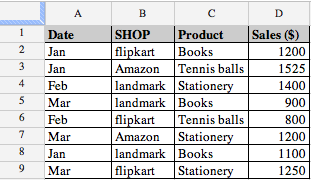We can easily count the number of Amazon with
=countif(B2:B9,”Amazon”)
which returns 2.
Similalrly, it is straight-forward to get the value of products sold through “Amazon”, using
=SUMIF(B2:B9,”Amazon”,D2:D9)
which gives 2725.
But supposing that we want a count of how many Amazon  sold in Jan, or the value of them? The number can be calculated with
=ArrayFormula(SUM(IF(B2:B9=”Amazon”,IF(A2:A9=”Jan”,1,0),0)))
which is an array formula. Similarly, the value is obtained with
=ArrayFormula(SUM(IF(B2:B9=”Amazon”,IF(A2:A9=”Jan”,D2:D9,0),0)))
which is also an array formula.

But as this page is about SUMPRODUCT, you would expect that we could use that function in this case, and we can. The solution for the number of Amazon sold in Jan using this function is
=ArrayFormula(SUMPRODUCT((B2:B9=”Amazon”)*(A2:A9=”Jan”)))
The value is obtained with
=ArrayFormula(SUMPRODUCT((B2:B9=”Amazon”)*(A2:A9=”Jan”)*(D2:D9)))
The * is being used as the AND operator, the formula is saying, where A2:A9 = Amazon AND B2:B9 = Jan, and where A2:A9 = Amazon AND B2:B9= Jan, multiplied by D2:D9.

This is relatively easy to use when there are multiple criteria.

We can see that the * is equivalent to AND in the formula, how this works is explained later, but supposing we want an OR condition. As a further extension of its use, we use the ‘+’ (plus) operator to count OR conditions, such as how many books sold were either
Flipkart or Landmark. The formula for this is
=ArrayFormula(SUMPRODUCT((B2:B9=”Flipkart”)+(B2:B9=”landmark”)))
which returns the result 6 as expected
So far, so good, in that we have a versatile function that can do any number of conditional tests, and has an inbuilt flexibility that provides extensibility.

SUMPRODUCT Explained

Understanding how SUMPRODUCT works helps to determine where to use it, how to can construct thus formula, and thus how it can be extended. below shows an example data set that we will use.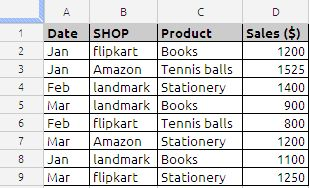In this example, the problem is to find how many Amazon with a date in Jan were sold. A2:A9 holds the Date, B2:B9 has the Shop, and D2:D9 has the number sold. The formula to get this result is
=ArrayFormula(SUMPRODUCT((B2:B9=”Amazon”)*(A2:A9=”Jan”)*(D2:D9)))
The first part of the formula (B2:B9=”Amazon”) checks the array of makes for a value of Amazon. This returns an array of TRUE/FALSE, in this case it is
{FALSE,TRUE,FALSE,FALSE,FALSE,TRUE,FALSE,FALSE}
Similarly, the categories are checked for the vale Date with (A2:A9=”Jan”). Again, this returns an array of TRUE/FALSE, or
{TRUE,TRUE,FALSE,FALSE,FALSE,FALSE,TRUE,FALSE}
And finally, the numbers are not checked but taken as is, that is (D2:D9), which returns an array of numbers
{1200,1525,1400,900,800,1200,1100,1250}
So now we have three arrays, two of TRUE/FALSE values, one of numbers. This is showm in below.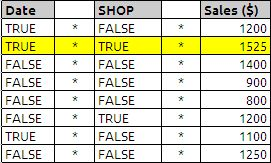And this is where it gets interesting.

SUMPRODUCT usually works on arrays of numbers, but we have arrays of TRUE/FALSE values as well as an array of numbers. By using the ‘*’ (multiply) operator, we can get numeric values that can be summed. ‘*’ has the effect of coercing these two arrays into a single array of 1/0 values. Multiplying TRUE by TRUE returns 1 (try it, enter =TRUE()*TRUE() in a cell and see the result), any other combination returns 0. Therefore, when both conditions are satisfied, we get a 1, whereas if any or both conditions are not satisfied, we get a 0. Multiplying the first array of TRUE/FALSE values by the second array of TRUE/FALSE values returns a composite array of 1/0 values, or

{0,1,0,0,0,0,0,0}*{1200,1525,1400,900,800,1200,1100,1250}
which will result in 1525

Similarly we can count the same
=ArrayFormula(SUMPRODUCT((B2:B9=”Amazon”)*(A2:A9=”Jan”)))
the arrays will be as show in the below example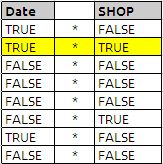and after executing the above formulas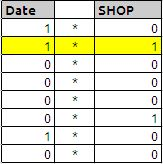and answer is highlighted in yellow colour which will result in value 1

If you have been able to follow this explanation all of the way through, it may have occurred to you that although we are using the SUMPRODUCT function, the ‘*’ operators have resolved the multiple arrays into a single composite array, leaving SUMPRODUCT to simply sum the members of that composite array, that is, there is no product. This is perfectly correct, and perfectly valid,SUMPRODUCT can work on a single array (put 1,2,3 in cells A1,A2,A3, and insert =SUMPRODUCT(A1:A3) in a cell, it returns 6 correctly). In reality, we only need the ‘*’ to coerce the arrays that are being tested for a particular condition, we do not need it for the array that is not subject to a conditional test. So we could also use

=ArrayFormula(SUMPRODUCT((B2:B9=”Amazon”)*(A2:A9=”Jan”)*(D2:D9)))

which does use the product aspect .

• Double uninary will not work in google docs spead sheets.
• When using the SUMPRODUCT function, all arrays must be the same size, as corresponding members of each array are multiplied by each other.
• When using the SUMPRODUCT function, do not use the open ranges it may cause errors

In a SUMPRODUCT function, the arrays being evaluated cannot be a mix of column and row ranges, they must all be columns, or all rows. However, the row data can be transposed to present it to SUMPRODUCT as columnar.

VLOOKUP

Vlookup is one of the most powerful functions in Spread sheets. So many people use Vlookup as a daily function, and is most useful in searching a table, looking for the same names, field or identifier and then spitting out an output based on that search criteria. It may sound difficult, but is quite easy

The VLOOKUP function retrieves an item from a table and returns it to a cell or formula.

The Syntax of the function is

VLOOKUP(search_criterion, array, index, sort_order)

The VLOOKUP:

1) Looks for a value in the leftmost column of a table

2) And then returns a value in the same row from a column you specify

3) By default, the table must be sorted in an ascending order

The VLOOKUP function has four arguments:

1) search_criterion: This is the items that the VLOOKUP looks at before it goes over to the table and looks it up. Once the VLOOKUP goes over to the table, it looks in the leftmost column to try and fine the value

2) array : is the table (database) that you are looking a value up in

3) index : is the number of the column that has the value you want to get and return back to the cell (the leftmost column of the table is considered 1). For this argument you can use the COLUMNS or ROWS functions to increment numbers.

4) sort_order : is a 0 when you are looking up an exact value (like a word) and 1 or omitted when you are looking up an approximate value (like in tax tables where you want to find the row that is equal to the lookup_value or greater than, but less than the next value in the leftmost column.

Notes:

• If index is less than 1, the VLookup function will return #VALUE!.
• If index is greater than the number of columns in table_array, the VLookup function will return #REF!.
• If you enter FALSE for the not_exact_match parameter and no exact match is found, then the VLookup function will return #N/A.

(1) The search_criterion argument is the “number that we are looking up.”.

• NOT case sensitive (TOM=tom=ToM=tOM)
• Cannot be longer than 255 characters.
• Can be a number, text, logical value, or a name or reference that refers to a one of these

(2) The array argument is the lookup table and can contain one or more columns.

• The first column is the lookup column
• The column with the values to return can be in any of the columns(1,2,3, and so on)
• If you are doing an approximate match, the first column must be sorted smallest to biggest.
• If you are doing an exact match, the first column does not need to be sorted.

(3) The Index argument is a number (1,2,3, and so on) that represented the column in the table_array that has the value to return to the cell.

(4) The sort_order argument tells the VLOOKUP function whether you are doing an approximate match or an exact match or an exact match when looking up a value.

If you are doing an approximate match, the first column must be sorted smallest to biggest(ascending)

If there are duplicates in the first column, VLOOKUP will choose the first one listed.

Now let see and understand the working of VLOOKUP function:

The sample data is taken from NSE (National Stock Exchange) of India web site, the first column is name of the company, second column in NSE stock symbol, third column is ISIN number and the  fourth column is face value of stock script.

the data used in this example available at the end of the post

suppose we want stock symbol of Gillette India Limited in cell B5 the formula will be

= VLOOKUP(A24,A2:D21,2,FALSE)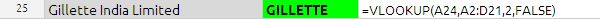in the above example search_criterion argument is A24 it is name of the stock we want to search in the data base or table.

array argument is table array which is from A2 to D21

index argument is the column index number in the above example it is  2 which stock symbol corresponding to script.

sort_order in the above example sort_order is FALSE because we want to exact match.

If there is no match for the search_criterion, the VLOOKUP spit the data one row above the nearest value.In the above example the APPLE COMPUTERS INC does not have any value in the table, but since we have put the Sort_order as TRUE which is nothing but approximate match. we have got the AMRUTANJAN.

In case of duplicate values the VLOOKUP function return the value in the first place.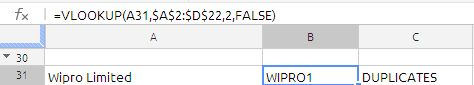in the data table if you see A21 and A22 has the same value, VLOOKUP function will return first value only. this is big constrain in the  VLOOKUP function.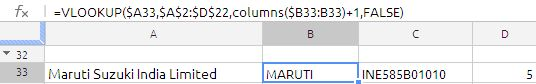the formula in cell B33 is

=VLOOKUP(\$A33,\$A\$2:\$D\$22,COLUMNS(\$B33:B33)+1,FALSE

If we drag it across we will get the data from  Symbol,ISIN, Face. the only logic inside the formula is correct use of relative reference and use of COLUMNS function which is column increment in the  above formula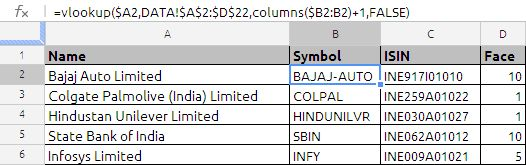in the above example the formula used in cell B2 is =VLOOKUP(\$A2,DATA!\$A\$2:\$D\$22,COLUMNS(\$B2:B2)+1,FALSE) drag it down and   cross

the above formula will fill the column B, C & D.

the above formula is traditional way of writing. But same can be modified in the google docs array formula as shown in the below example: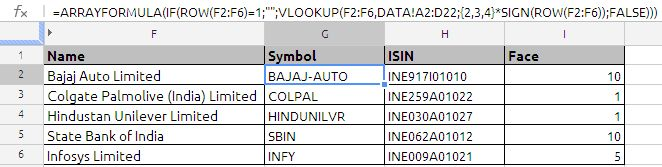this is single most efficient, no dragging is required.

the formula used in cell G2 is =ARRAYFORMULA(IF(ROW(F2:F6)=1;””;VLOOKUP(F2:F6,DATA!A2:D22;{2,3,4}*SIGN(ROW(F2:F6));FALSE)))

most noticeable point is use of {2,3,4} which is array of column numbers which we want to extract. SIGN function is used to convert the true /false to 1 and 0

VLOOKUP is more versatile, most used function, i am only trying to explain with basic futures only.

.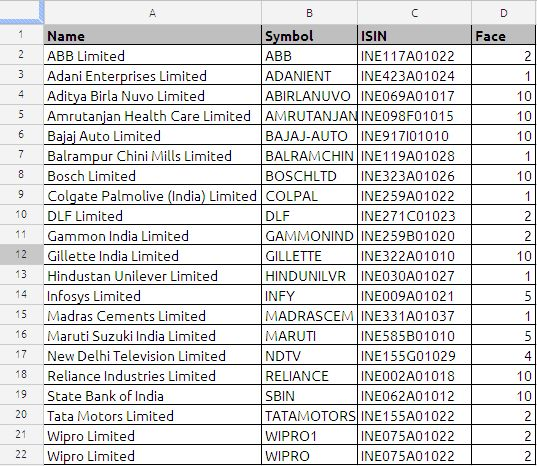PMT function

The PMT function returns the fixed periodic payment for a loan or annuity based on a series of regular periodic cash flows (payments of a constant amount and all cash flows at constant intervals) and a fixed interest rate.

the syntax of PMT function is as follows:
PMT(rate, NPER, PV, FV, type)

rate:  The interest rate per period. periodic-rate is a number value and is either entered as a decimal (for example, 0.08) or with a percent sign (for example, 8%). If the value given is in 8% per annual to convert this into monthly rate we have divide this value by 12

NPER:  The number of periods. NPER is a number value and must be
greater than or equal to 0.

PV:  The value of the initial investment, or the amount of the loan or annuity. present-value is a number value. At time 0, an amount received is a positive amount and an amount invested is a negative amount. For example, it could be an amount borrowed (positive) or the initial payment made on an annuity contract (negative).

Note: Suppose if we are calculating the EMI (equated monthly installment) for the amount borrowed. the PMT function will spit out a negative value (-ve) because this much amount is going out of our pocket to the lender. To avoid this native values in PMT function we may enter this value as minus followed by value

FV:  An optional argument that represents the value of the investment or remaining cash value of the annuity (positive amount), or the remaining loan balance (negative amount), after the final payment. future-value is a number value. At the end of the investment period, an amount received is a positive amount and an amount invested is a negative amount. For example, It could be the balloon payment due on a loan (negative) or the remaining value of an annuity contract (positive). If omitted, it is assumed to be 0.

Note: while calculative the EMI normally we ignore the this value.

type:  An optional argument that specifies whether payments are due at the beginning or end of each period. Most mortgage and other loans require the first payment at the end of the first period (0), which is the default. Most lease and rent payments, and some other types of payments, are due at the beginning of each period (1).

end (0 or omitted):  Payments are due at the end of each period.
beginning (1):  Payments are due at the beginning of each period.

Now lets look at an example:
We have borrowed \$1,00,000 at an interest rate of 12% p.a and repayable in 2 years the amortisation table as mentioned below:

lets look at the formulas:
in cell  C7
=PMT(\$B\$2/12,\$B\$3*12,-\$B\$1)  drag it down till cell C30 or
use the google docs single  formula to populate to cell C7 TO C30
=ArrayFormula(if(len(A7:A30);ROUND(PMT(\$B\$2/12,\$B\$3*12,-\$B\$1),2);iferror(1/0)))

Now we breakup the formula
The first argument is rate we have used \$B\$2/12 since our rate of interest is give in annual we have to convert this to  monthly rate.

The second argument is  NPER which is number of periods, here in this example we are repaying the principal within the 2 years so we are multiplying the 2 with number of period which 24

The third argument is PV the present value of the loan is 100000 we have to precede this with minus symbol so that the PMT function will spit the positive value

The fourth argument is FV we have ignored this value

The fifth argument is TYPE this arguments also we have ignored this means we are making the payment at the end of the month

to avoid more than two decimals in the value we can warp it with round function as mentioned below
=ROUND(PMT(\$B\$2/12,\$B\$3*12,-\$B\$1),2)

the formula in cell D7 is
=ROUND(\$B7*\$B\$2/12,2) and drag it down  to calculated interest in the EMI or
use the google docs array formula
=ArrayFormula(if(len(A7:A30);ROUND(B7:B30*\$B\$2/12,2);iferror(1/0)))

the formula in  cell E7 is
=ROUND(B7-C7+D7,2)
to calculate the closing principal outstanding

To calculate the interest in the first 12 months without preparing the table we can use the following formula

=ROUND(ArrayFormula(SUM(IPMT(\$B\$2/12,ROW(1:12),24,-B1))),2)
which will give you the result of 9469.72
you can verify the same by adding interest column first 12 rows from D7 TO D18
1000 + 962.93 + 925.48 +887.66 + 849.47 + 810.89 + 771.92 + 732.57 + 692.82 + 652.68 + 612.13 + 571.18 = 9469.72

similar for next 12 months the formula will be
=ROUND(ArrayFormula(SUM(IPMT(\$B\$2/12,ROW(13:24),24,-B1))),2) the result will be 3506.61

Similarly to calculate the Principal repayment for the first 12 months the formula will be
=ROUND(ArrayFormula(SUM(PPMT(\$B\$2/12,ROW(1:12),24,-B1))),2) the result will be 47018.44

for the second 12 month i.e. from month 13 to 24 the principal repayment will be
=ROUND(ArrayFormula(SUM(PPMT(\$B\$2/12,ROW(13:24),24,-B1))),2) the result will be 52981.56

we can verify this by adding the above two (i.e.47018.44 + 52981.56) we will get 100000 which is same as principal what we have borrowed from lender.

Example data